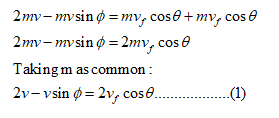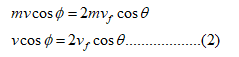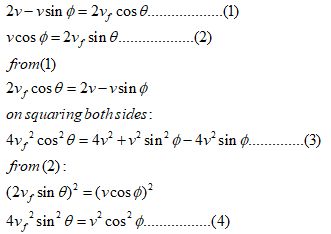Question
2 views

Collision at an Angle

To apply conservation of linear momentum in an inelastic collision.  Two cars, both of mass m, collide and stick together. Prior to the collision, one car had been traveling north at a speed 2v, while the second was traveling in a southeastern direction at an angle ϕ with respect to the east-west direction and at a speed v. After the collision, the two-car system travels in a northeastern direction at an angle θ with respect to the north-south direction and at a speed v final.

Find v final, the speed of the joined cars after the collision.

check_circle

Step 1

Given:

The mass of each car = m kg

The one car travelling north has speed = 2v m/s

The speed of another car = v m/s

The final speed = vm/s

Step 2

Solution:

To determine the final velocity, we will apply the momentum conservation along the North directionNow applying the momentum conservation in east direction:Step 3

On solving (1) and (2) we get:

We will be squaring and adding both equations to get the result:...

### Want to see the full answer?

See Solution

#### Want to see this answer and more?

Solutions are written by subject experts who are available 24/7. Questions are typically answered within 1 hour.*

See Solution
*Response times may vary by subject and question.
Tagged in